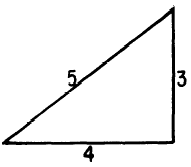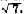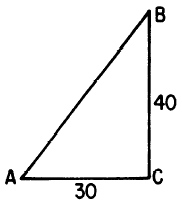Custom SearchTHE 3-4-5 TRIANGLE The triangle shown in figure 19-14 has its sides in the ratio 3 to 4 to 5. Any triangle with its sides in this ratio is a right triangle. It is a common error to assume that a triangle is a 3-4-5 type because two sides are known to be in the ratio 3 to 4, or perhaps 4 to 5. Figure 19-15 shows two examples of triangles which happen to have two of their sides in the stated ratio, but not the third side. ThisFigure 19-13.-Finding unknown parts in a 45-90 triangle.Figure 19-14.-A 3-4-5 triangle.Figure 19-15.-Triangles which may be mistaken for 3-4-5 triangles.  can be because the triangle is not a right triangle, as in figure 19-15 (A). On the other hand, even though the triangle is a right triangle its longest side may be the 4-unit side, in which case the third side cannot be 5 units long. (See fig. 19-15 (B).) It is interesting to note that the third side in figure 19-15 (B) isThis is a very unusual coincidence, in which one side of a right triangle is the square root of the sum of the other two sides. Related to the basic 3-4-5 triangle are all triangles whose sides are in the ratio 3 to 4 to 5 but are longer (proportionately) than these basic lengths. For example, the triangle pictured in figure 19-6 is a 3-4 -5 triangle.Figure 19-16.-Triangle with sides which are multiples of 3, 4, and 5. The 3-4-5 triangle is very useful in calculations of distance. If the data can be adapted to fit a 3-4-5 configuration, no tables or calculation of square root (Pythagorean Theorem) are needed. EXAMPLE: An observer at the top of a 40-foot vertical tower knows that the base of the tower is 30 feet from a target on the ground. How does he calculate his slant range (direct line of sight) from the target? SOLUTION: Figure 19-17 shows that the desired length, AB, is the hypotenuse of a right triangle whose shorter sides are 30 feet and 40 feet long. Since these sides are in the ratio 3 to 4 and angle C is 90) the triangle is a 3-4-5 triangle. Therefore, side AB represents the 5-unit side of the triangle. The ratio 30 to 40 to 50 is equivalent to 3-4-5, and thus side AB is 50 units long. Practice problems. Without reference to tables or to the rule of Pythagoras, solve the following problems: 1. An observer is at the top of a 30-foot vertical tower. Calculate his slant range from a target on the ground which is 40 feet from the base of the tower.Figure 19-17.-Solving problems with a 3-4-5 triangle. 2. A guy wire 15 feet long is stretched from the top of a pole to a point on the ground 9 feet from the base of the pole. Calculate the height of the pole. Answers: 1. 50 feet  2. 12 feetIntegrated Publishing, Inc. - A (SDVOSB) Service Disabled Veteran Owned Small Business